# 2. The joint pdf of random variables X and Y is given by f(x.y) k if...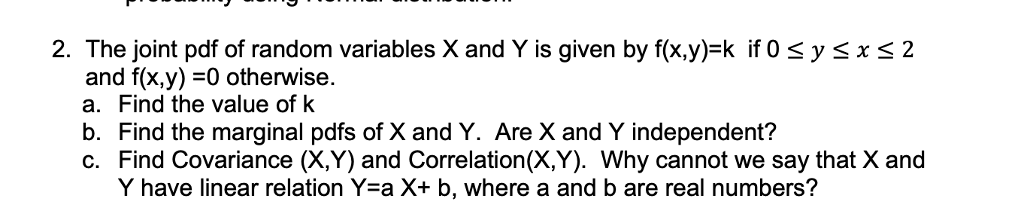2. The joint pdf of random variables X and Y is given by f(x.y) k if 0 sysxs2 and f(x,y)-0 otherwise. a. Find the value of k b. Find the marginal pdfs of X and Y. Are X and Y independent? c. Find Covariance (X,Y) and Correlation(X,Y). Why cannot we say that X and Y have linear relation Yea X+ b, where a and b are real numbers?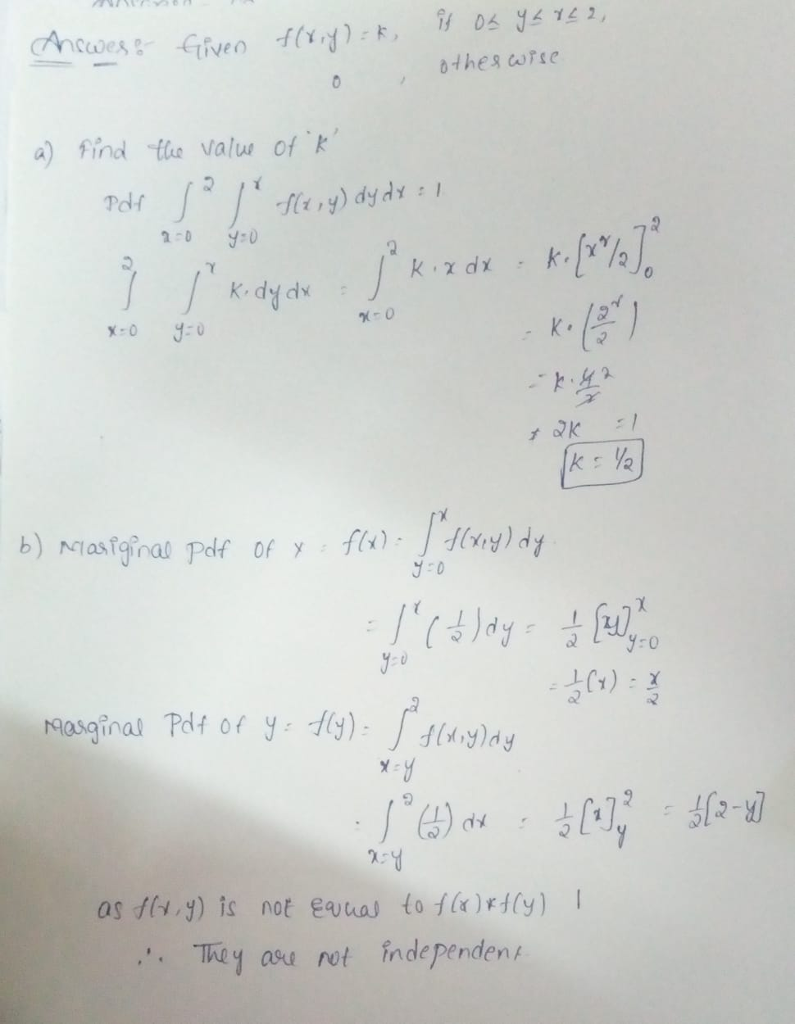#### Earn Coin

Coins can be redeemed for fabulous gifts.

Similar Homework Help Questions
• ### The joint pdf of random variables X and Y is given by f(x.y)-k if 0 s...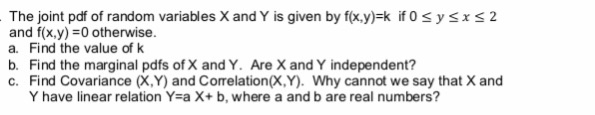The joint pdf of random variables X and Y is given by f(x.y)-k if 0 s y sx s 2 and f(x,y) =0 otherwise. a. Find the value of k b. Find the marginal pdfs of X and Y. Are X and Y independent? c. Find Covariance (X,Y) and Correlation(X,Y). Why cannot we say that X and Y have linear relation Y-a X+ b, where a and b are real numbers?

• ### 2. Let X and Y be continuous random variables with joint pdf fx.y (x. y)- 3x,...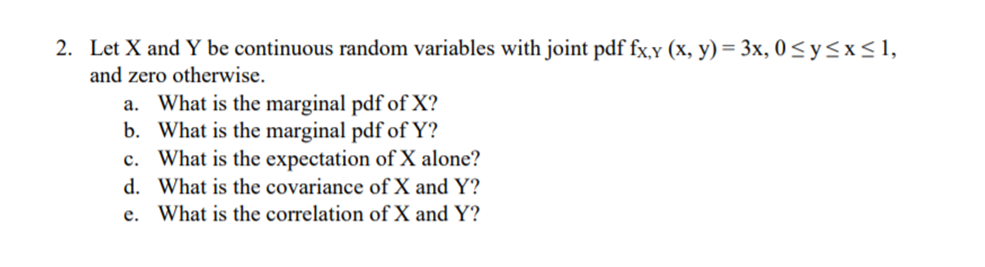2. Let X and Y be continuous random variables with joint pdf fx.y (x. y)- 3x, 0 Syx, and zero otherwise. a. b. c. d. e. What is the marginal pdf of X? What is the marginal pdf of Y? What is the expectation of X alone? What is the covariance of X and Y? What is the correlation of X and Y?

• ### 2. Let X and Y be continuous random variables with joint probability density function fx,y(x,y) 0, otherwise (a) Compute the value of k that will make f(x, y) a legitimate joint probability density f...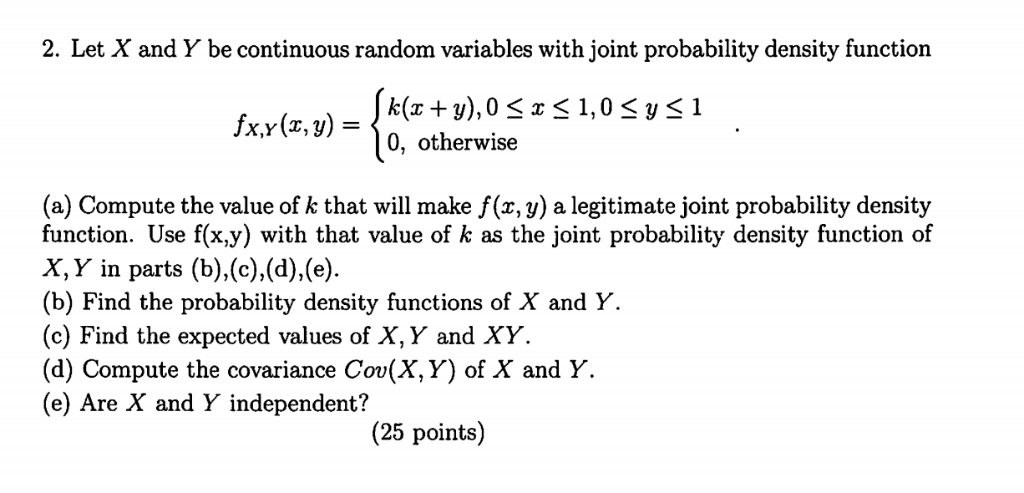2. Let X and Y be continuous random variables with joint probability density function fx,y(x,y) 0, otherwise (a) Compute the value of k that will make f(x, y) a legitimate joint probability density function. Use f(x.y) with that value of k as the joint probability density function of X, Y in parts (b),(c).(d),(e (b) Find the probability density functions of X and Y. (c) Find the expected values of X, Y and XY (d) Compute the covariance Cov(X,Y) of X...

• ### 2. Let the random variables X and Y have the joint PDF given below: (a) Find P(X + Y ≤ 2). (b) Find the marginal PDFs o...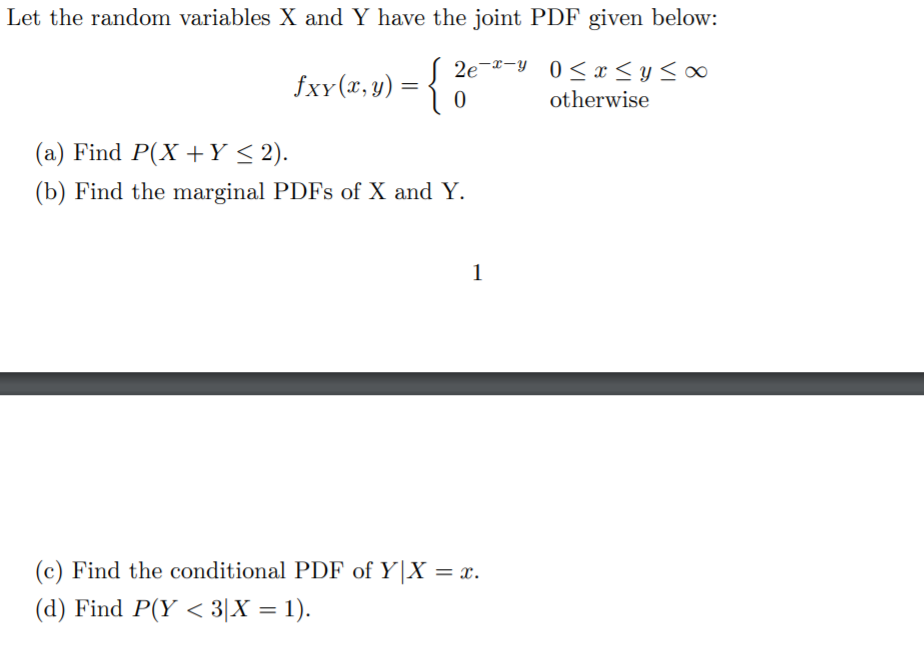2. Let the random variables X and Y have the joint PDF given below: (a) Find P(X + Y ≤ 2). (b) Find the marginal PDFs of X and Y. (c) Find the conditional PDF of Y |X = x. (d) Find P(Y < 3|X = 1). Let the random variables X and Y have the joint PDF given below: 2e -0 < y < 00 xY(,) otherwise 0 (a) Find P(XY < 2) (b) Find the marginal PDFs of...

• ### Let X and Y be continuous random variables with joint pdf fx.v (x, y)-3x, OSysx<1, and...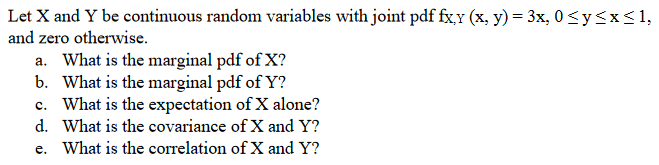Let X and Y be continuous random variables with joint pdf fx.v (x, y)-3x, OSysx<1, and zero otherwise. a. b. c. d. e. What is the marginal pdf of X? What is the marginal pdf of Y? What is the expectation of X alone? What is the covariance of X and Y? What is the correlation of X and Y?

• ### Let X and Y be continuous random variables with joint pdf fx y (x, y)-3x, 0...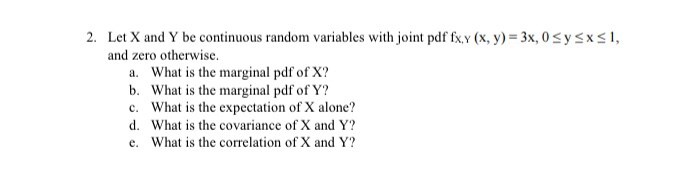Let X and Y be continuous random variables with joint pdf fx y (x, y)-3x, 0 Sy and zero otherwise. 2. sx, a. What is the marginal pdf of X? b. What is the marginal pdf of Y? c. What is the expectation of X alone? d. What is the covariance of X and Y? e. What is the correlation of X and Y?

• ### 2. Let the random variables X and Y have the joint PDF given below: S 2e-2-Y...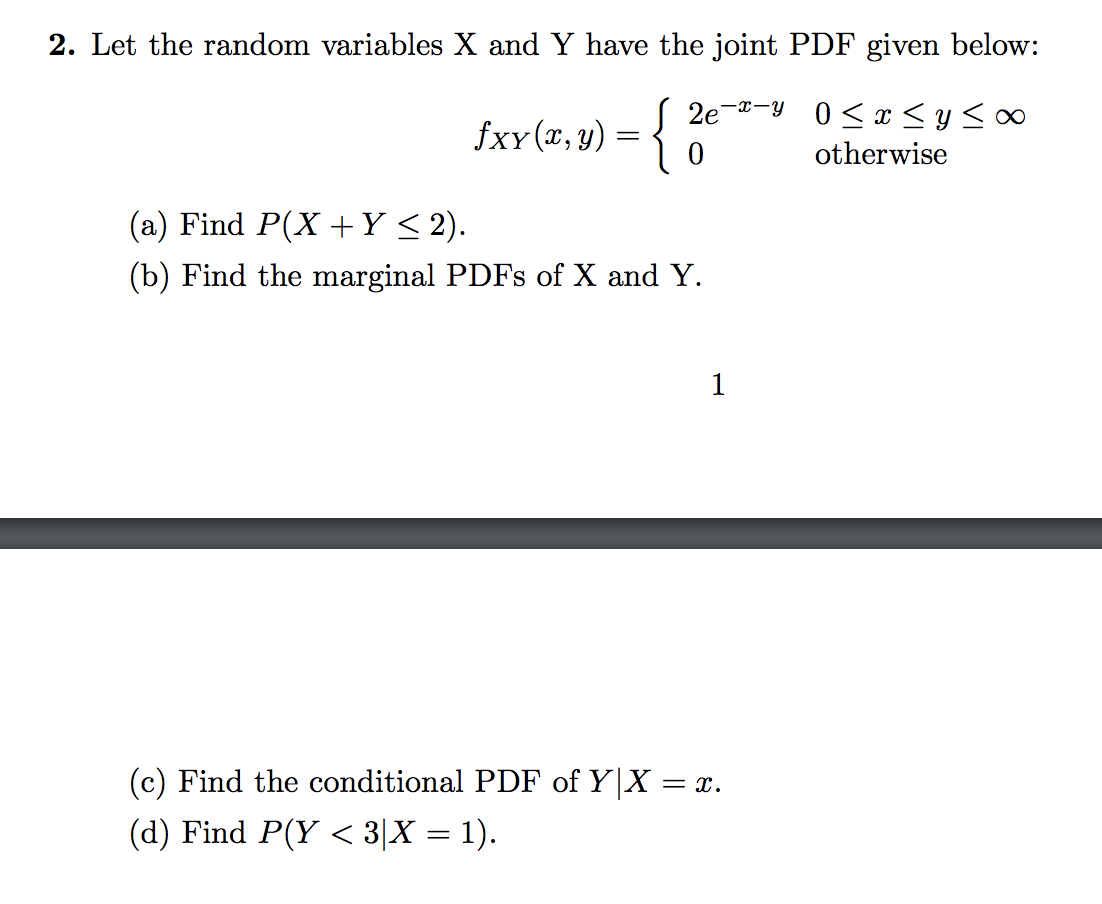2. Let the random variables X and Y have the joint PDF given below: S 2e-2-Y 0 < x < y < fxy(x,y) = { 0 otherwise (a) Find P(X+Y < 2). (b) Find the marginal PDFs of X and Y. (c) Find the conditional PDF of Y|X = r. (d) Find P(Y <3|X = 1).

• ### Problem 2 - Three Continuous Random Variables Suppose X,Y,Z have joint pdf given by fx,YZ(xgz) = k xyz if 0 S\$ 1,0 rS 1,0 25 1 ) and fxyZ(x,y,z) = 0, otherwise. (a) Find k so that fxyz(x.yz) is a gen...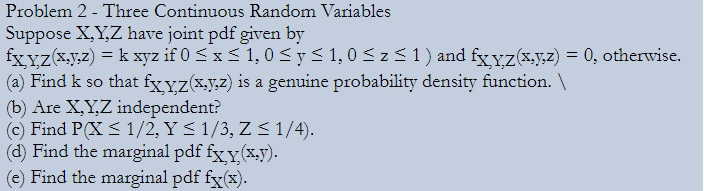Problem 2 - Three Continuous Random Variables Suppose X,Y,Z have joint pdf given by fx,YZ(xgz) = k xyz if 0 S\$ 1,0 rS 1,0 25 1 ) and fxyZ(x,y,z) = 0, otherwise. (a) Find k so that fxyz(x.yz) is a genuine probability density function. (b) Are X,Y,Z independent? (c) Find PXs 1/2, Y s 1/3, Z s1/4). (d) Find the marginal pdf fxy(x.y). (e) Find the marginal pdf fx(x). Problem 2 - Three Continuous Random Variables Suppose X,Y,Z have joint...

• ### Suppose the joint pdf of random variables X and Y is f(x,y) = c/x, 0 <...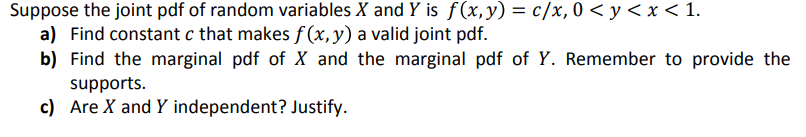Suppose the joint pdf of random variables X and Y is f(x,y) = c/x, 0 < y < x < 1. a) Find constant c that makes f (x, y) a valid joint pdf. b) Find the marginal pdf of X and the marginal pdf of Y. Remember to provide the supports c) Are X and Y independent? Justify

• ### 2. Let the random variables X and Y have the joint PDF given below: 2e -y...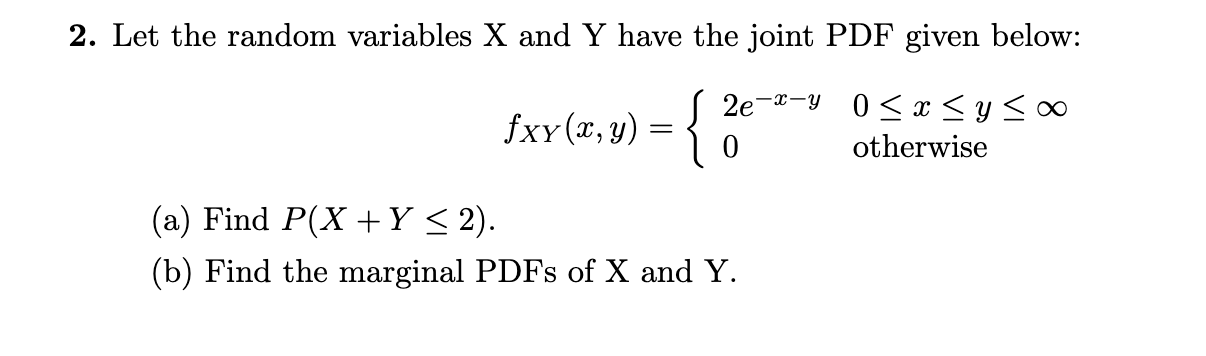2. Let the random variables X and Y have the joint PDF given below: 2e -y 0 xyo0 fxy (x, y) otherwise 0 (a) Find P(X Y < 2) (b) Find the marginal PDFs of X and Y (c) Find the conditional PDF of Y X x (d) Find P(Y< 3|X = 1)